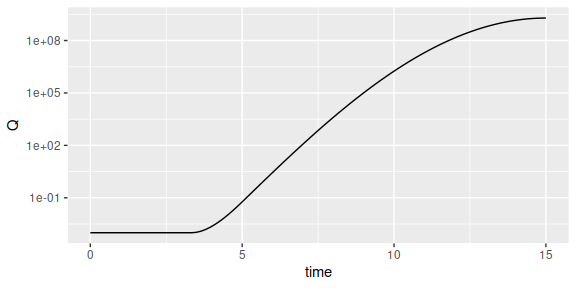# Advanced plotting options in biogrowth

library(biogrowth)
library(tidyverse)
library(cowplot)

This vignette gives further detail on how to use the functions in biogrowth to prepare publication ready figures. For details on how to use the base functions of the package, please check the vignettes for model fitting and growth predictions.

The functions in biogrowth use cowplot::theme_cowplot as default, that has a nice clean theme and could be used in a publication as is. However, more control over formatting options can be needed in order to standardize plots or when preparing figures for a specific journal.

Moreover, every plot is based on ggplot2, and therefore the plots can be manipulated in the same way. In order to ease manipulation, plotting methods in biogrowth include arguments to control the aesthetics that would often would within the “geom_” definition (such as colour, size or linetype).

As an illustration for this, we will use a growth model fitted to data under dynamic environmental conditions.

data("example_dynamic_growth")
data("example_env_conditions")

sec_models <- list(temperature = "CPM", aw = "CPM")

known_pars <- list(Nmax = 1e4,  # Primary model
N0 = 1e0, Q0 = 1e-3,  # Initial values of the primary model
mu_opt = 4, # mu_opt of the gamma model
temperature_n = 1,  # Secondary model for temperature
aw_xmax = 1, aw_xmin = .9, aw_n = 1  # Secondary model for water activity
)
my_start <- list(temperature_xmin = 25, temperature_xopt = 35,
temperature_xmax = 40, aw_xopt = .95)

my_model <- fit_growth(example_dynamic_growth,
sec_models,
my_start, known_pars,
environment = "dynamic",
env_conditions = example_env_conditions
) 

By default, the S3 plot() methods use the cowplot::theme_cowplot

plot(my_model)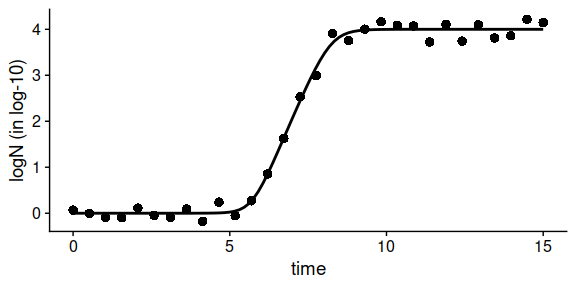As mentioned, the plot methods include a large list of additional arguments that can be used to edit the aesthetics of the plot. A whole list of arguments is available from the class documentation (e.g. accessible by typing ?GrowthFit in the console). In the case of plot.GrowthFit(), the plot method includes the following arguments:

• add_factor
• line_col
• line_size
• line_type
• point_col
• point_size
• point_shape
• ylims
• label_y1
• label_y2
• label_x
• line_col2
• line_size2
• line_type2

This provides plenty of options to edit the aesthetics of the plot. For instance:

plot(my_model,
line_col = "red",
line_size = 1,
line_type  = "dashed",
label_y1 = "Population size (log-millions)",
label_x = "Time (years)",
point_size = 3,
point_shape = 1,
point_col = "darkgrey")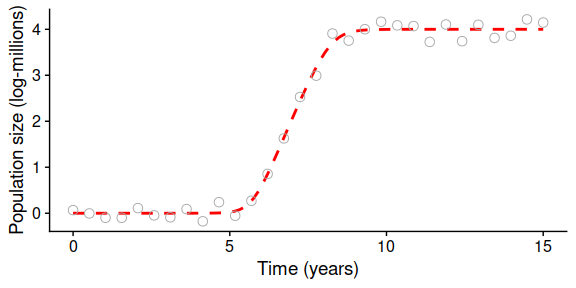Note that the plot function returns an instance of ggplot. This allows further editing of the plot using layers with the functions included in ggplot2. This provides plenty of options to edit the plot

plot(my_model,
line_col = "red",
line_size = 1,
line_type  = "dashed",
label_y1 = "Population size (log-millions)",
label_x = "Time (years)",
point_size = 3,
point_shape = 1,
point_col = "darkgrey") +
theme_gray() +
theme(axis.title = element_text(colour = "green", size = 14))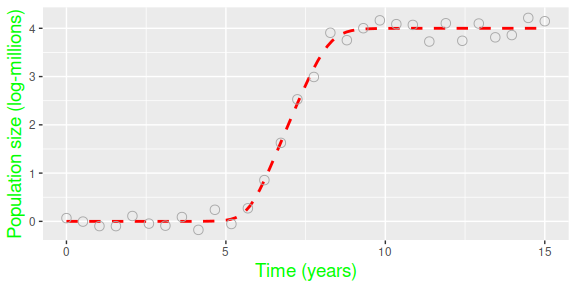In some cases, the automatic scaling that ggplot2 uses might not be optimal. We can use the coord_cartesian() function to change the limits of the x- and y-axis

plot(my_model) +
coord_cartesian(xlim = c(5, 10), ylim = c(0, 4)) # changing the axis limits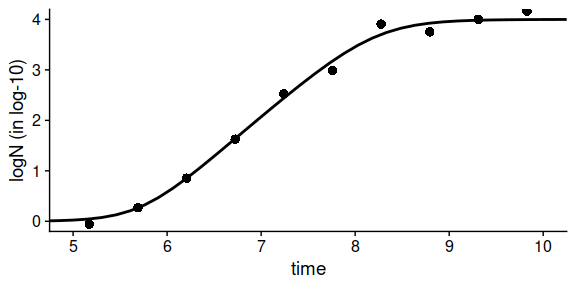## Combining plots into subplots

The plot_grid() function from cowplot provides a convenient way to combine different plots into a grid. For instance,

p1 <- plot(my_model, add_factor = "temperature")
p2 <- plot(my_model, add_factor = "aw")
plot_grid(p1, p2, labels = "AUTO")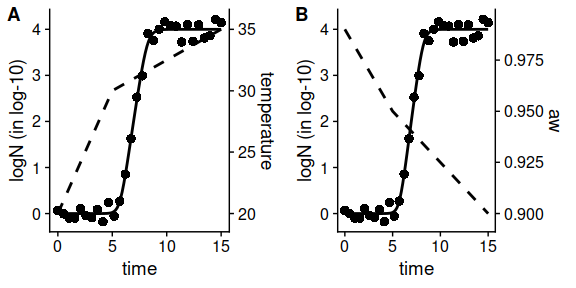## Saving and reshaping plots

ggsave() will automatically save the last plot to a specified location. It needs a filename as a string, for instance “static_prediction.pdf” to save the figure as a pdf. It also needs a location to save to (defaults to the working directory), and optionally the user can set dimentions and units.

# We save the plot as p1, notice that it does not get drawn now
p1 <- plot(my_model, line_col = "red")

# We save P1 as .pdf, as a 20x 20 cm square
# ggsave("static_prediction.pdf", p1, width = 20, height = 20, units = "cm")

# Full manual control

The classes GrowthFit, GrowthPrediction and GlobalGrowthFit are a subclass of list. This provides simple access to several attributes of the model. For instance, the the entry best_prediction of an instance of GrowthFit includes an instance of GrowthPrediction with the fitted model.

my_model$best_prediction #> Growth prediction under dynamic environmental conditions #> #> Environmental factors included: temperature, aw #> #> Parameters of the Baranyi primary model: #> mu_opt Nmax N0 Q0 #> 4e+00 1e+04 1e+00 1e-03 #> #> Parameter mu defined in log-10 scale #> #> Population size defined in log-10 scale #> #> Secondary model for temperature: #> xmin xopt xmax n #> "26.6667032815958" "38.8653415561503" "70.3684359647904" "1" #> model #> "CPM" #> #> Secondary model for aw: #> xmin xopt xmax n #> "0.9" "0.985185030452571" "1" "1" #> model #> "CPM" In a similar way, the simulation entry of this instance includes a tibble with the model simulation head(my_model$best_prediction$simulation) #> # A tibble: 6 × 4 #> time Q N logN #> <dbl> <dbl> <dbl> <dbl> #> 1 0 0.001 1 0 #> 2 0.152 0.001 1 0 #> 3 0.303 0.001 1 0 #> 4 0.455 0.001 1 0 #> 5 0.606 0.001 1 0 #> 6 0.758 0.001 1 0 This allows making plots directly using this data using ggplot2 (or similar packages) ggplot(my_model$best_prediction\$simulation) +
geom_line(aes(x = time, y = Q)) +
scale_y_log10()